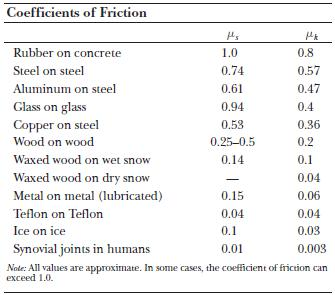# Friction

Friction is a dissipative force. It does not conserve energy within a system, but rather disperses it into the surroundings. It is also a resistive force that is present between any two objects that are in contact. It always acts in the opposite direction to motion.

The amount of friction between objects depends, in general, on 2 things:

1. The kinds of surfaces of the objects
2. The force pressing the surfaces together

The causes of friction may be complicated: Consider an example of a book lying on a table.

The book’s weight slightly deforms the surface of the table along with that of the book. A plowing force is required to move the book over these deformations. The irregularities on both surfaces tend to interlock (like mountains and valleys fitting together) and offer resistance to the sliding of the book. During this process, tiny particles are torn from one surface and become imbedded in the other.

Although polishing will reduce friction from the above causes, there is a limit to the amount friction can be reduced by polishing. At some point, there will actually be an increase in the friction. This seems to be caused by forces of attraction between the molecules of the substances.

Friction may be desirable . For instance, without friction, you could not sit in your chair you would slide out. Without friction, we could not walk. Friction between our feet and the floor (or ground) allows us to move forward. If there were no friction in a car, we could not stop it. We could not even go around curves on the road without friction.

However, there are also many instances in which friction is not desirable . We put oil in cars to reduce the friction between the moving components in the engine. Machines, hinges, locks, etc., are oiled or otherwise lubricated to reduce friction.

Friction experiments are not at all difficult to perform, but the results are not always easy to compile. There are so many variables. Conversely, it is all but impossible to perform frictionless experiments. When objects are in contact, there is friction. We have ways in physics to get around much of this, using air tracks, etc., but there is still friction present.

Because of this, we have something called the coefficient of friction which must be taken into account.

The coefficient of friction (unless otherwise stated, it will be the coefficient of sliding friction) is the ratio of the force of sliding friction to the normal (perpendicular) force pressing the surfaces together.

Note that the symbol for the coefficient of sliding friction is (). There are 4 kinds of friction: static (stationary), sliding, rolling, and fluid. As a rule, general physics is only concerned with static and sliding friction. (Sliding friction is often referred to as kinetic friction. Kinetic friction = fk)

Static friction fs – opposes the impending relative motion between two objects.

Kinetic friction fk – opposes the relative sliding motions that actually do occur.Note that the static friction is normally greater than the kinetic friction.

For any object moving over a horizontal surface under the influence of an applied force F, the Net force (horizontally, causing motion) = [F(component in horizontal direction) – fk]

FN normal force; perpendicular to surface

Ff force of friction; opposite to motion (includes f sub s – static friction and f sub k – kinetic friction)

FW weight of object; straight down into Earth

FP force parallel to surface

Fa applied force

For an object moving down (i.e., in an elevator), the upward force is the pull of the elevator’s cable; the downward force is the pull of gravity; if the elevator is in motion, the acceleration of the elevator a is directed either up against gravity or down with gravity.

For connected objects (frictionless string passing over a pulley, one object on table, one hanging from pulley), both objects have an acceleration a. Also involved are a tension T in the string and the kinetic friction f sub k between the object on the table and the table. The sum of the forces acting on the block on the table in the horizontal direction is

ΣFx = T – fk

The sum of the forces acting on the block on the table in the vertical direction is

ΣFy = N – m1g where m1 = block on table

Remember that fk = µN where N = normal force

For the block hanging down, the sum of the forces in the vertical direction is

ΣFy = m2g – T where m2 = mass of block hanging down

Setting both equations = ma (using Newton’s 2nd law), and combining the equations, we get

a = (m2g – µkm1g) / (m1 + m2)

We can use this to solve for T

This is the system approach to solving problems involving frictional forces.

(source)